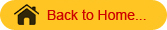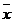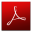## STAM101 :: Lecture 08 :: Sampling-basic concepts#### Sampling vs Complete enumeration parameter and statistic-sampling methods-simple random sampling and stratified random sampling

Population (Universe)
Population means aggregate of all possible units. It need not be human population. It may be population of plants, population of insects, population of fruits, etc.

Finite population
When the number of observation can be counted and is definite, it is known as finite population

• No. of plants in a plot.
• No. of farmers in a village.
• All the fields under a specified crop.

Infinite population
When the number of units in a population is innumerably large, that we cannot count all of them, it is known as infinite population.

• The plant population in a region.
• The population of insects in a region.

Frame
A list of all units of a population is known as frame.
Parameter
A summary measure that describes any given characteristic of the population is known as parameter. Population are described in terms of certain measures like mean, standard deviation etc. These measures of the population are called parameter and are usually denoted by Greek letters. For example, population mean is denoted by m, standard deviation by s and variance by s2 .
Sample
A portion or small number of unit of the total population is known as sample.

• All the farmers in a village(population) and a few farmers(sample)
• All plants in a plot is a population of plants.
• A small number of plants selected out of that population is a sample of plants.

Statistic
A summary measure that describes the characteristic of the sample is known as statisitic. Thus sample mean, sample standard deviation etc is statistic. The statistic is usually denoted by roman letter.- sample mean
s – standard deviation
The statistic is a random variable because it varies from sample to sample.
Sampling
The method of selecting samples from a population is known as sampling.
Sampling technique
There are two ways in which the information is collected during statistical survey. They are

• Census survey
• Sampling survey

Census
It is also known as population survey and complete enumeration survey. Under census survey the information are collected from each and every unit of the population or universe.
Sample survey
A sample is a part of the population. Information are collected from only a few units of a population and not from all the units. Such a survey is known as sample survey.
Sampling technique is universal in nature, consciously or unconsciously it is adopted in every day life.
For eg.

• A handful of rice is examined before buying a sack.
• We taste one or two fruits before buying a bunch of grapes.
• To measure root length of plants only a portion of plants are selected from a plot.

Need for sampling
The sampling methods have been extensively used for a variety of purposes and in great diversity of situations.
In practice it may not be possible to collected information on all units of a population due to various reasons such as

• Lack of resources in terms of money, personnel and equipment.
• The experimentation may be destructive in nature. Eg- finding out the germination percentage of seed material or in evaluating the efficiency of an insecticide the experimentation is destructive.
• The data may be wasteful if they are not collected within a time limit. The census survey will take longer time as compared to the sample survey. Hence for getting quick results sampling is preferred.  Moreover a sample survey will be less costly than complete enumeration.
• Sampling remains the only way when population contains infinitely many number of units.
• Greater accuracy.

Sampling methods
The various methods of sampling can be grouped under
1) Probability sampling or random sampling
2) Non-probability sampling or non random sampling
Random sampling
Under this method, every unit of the population at any stage has equal chance (or) each unit is drawn with known probability. It helps to estimate the mean, variance etc of the population.

Random Samples

Under probability sampling there are two procedures

• Sampling with replacement (SWR)
• Sampling without replacement (SWOR)

When the successive draws are made with placing back the units selected in the preceding draws, it is known as sampling with replacement. When such replacement is not made it is known as sampling without replacement.
When the population is finite sampling with replacement is adopted otherwise SWOR is adopted.
Mainly there are many kinds of random sampling. Some of them are.

• Simple Random Sampling
• Systematic Random Sampling
• Stratified Random Sampling
• Cluster Sampling

Simple Random sampling (SRS)
The basic probability sampling method is the simple random sampling. It is the simplest of all the probability sampling methods. It is used when the population is homogeneous.
When the units of the sample are drawn independently with equal probabilities. The sampling method is known as Simple Random Sampling (SRS). Thus if the population consists of N units, the probability of selecting any unit is 1/N.
A theoretical definition of SRS is as follows
Suppose we draw a sample of size n from a population of size N. There are NCn possible samples of size n. If all possible samples have an equal probability 1/NCn of being drawn, the sampling is said be simple random sampling.
There are two methods in SRS

• Lottery method
• Random no. table method

Lottery method
This is most popular method and simplest method. In this method all the items of the universe are numbered on separate slips of paper of same size, shape and color. They are folded and mixed up in a drum or a box or a container. A blindfold selection is made. Required number of slips is selected for the desired sample size. The selection of items thus depends on chance.
For example, if we want to select 5 plants out of 50 plants in a plot, we number the 50 plants first. We write the numbers from 1-50 on slips of the same size, role them and mix them. Then we make a blindfold selection of 5 plants. This method is also called unrestricted random sampling because units are selected from the population without any restriction. This method is mostly used in lottery draws. If the population is infinite, this method is inapplicable. There is a lot of possibility of personal prejudice if the size and shape of the slips are not identical.
Random number table method
As the lottery method cannot be used when the population is infinite, the alternative method is using of table of random numbers.
There are several standard tables of random numbers. But the credit for this technique goes to Prof. LHC. Tippet (1927). The random number table consists of 10,400 four-figured numbers. There are various other random numbers. They are fishers and Yates (19380 comprising of 15,000 digits arranged in twos. Kendall and B.B Smith (1939) consisting of 1, 00,000 numbers grouped in 25,000 sets of 4 digit random numbers, Rand corporation (1955) consisting of 2, 00,000 random numbers of 5 digits each etc.,
Merits

• There is less chance for personal bias.
• Sampling error can be measured.
• This method is economical as it saves time, money and labour.

Demerits

• It cannot be applied if the population is heterogeneous.
• This requires a complete list of the population but such up-to-date lists are not available in many enquires.
• If the size of the sample is small, then it will not be a representative of the population.

Stratified Sampling
When the population is heterogeneous with respect to the characteristic in which we are interested, we adopt stratified sampling.
When the heterogeneous population is divided into homogenous sub-population, the sub-populations are called strata. From each stratum a separate sample is selected using simple random sampling. This sampling method is known as stratified sampling.
We may stratify by size of farm, type of crop, soil type, etc.
The number of units to be selected may be uniform in all strata (or) may vary from stratum to stratum.
There are four types of allocation of strata

• Equal allocation
• Proportional allocation
• Neyman’s allocation
• Optimum allocation

If the number of units to be selected is uniform in all strata it is known as equal allocation of samples.
If the number of units to be selected from a stratum is proportional to the size of the stratum, it is known as proportional allocation of samples.
When the cost per unit varies from stratum to stratum, it is known as optimum allocation.
When the costs for different strata are equal, it is known as Neyman’s allocation.
Merits

• It is more representative.
• It ensures greater accuracy.
• It is easy to administrate as the universe is sub-divided.

Demerits

• To divide the population into homogeneous strata, it requires more money, time and statistical experience which is a difficult one.
• If proper stratification is not done, the sample will have an effect of bias.

Questions

1. If each and every unit of population has equal chance of being included in the sample,
it is known as
(a) Restricted sampling                    (b) Purposive sampling
(c) Simple random sampling           (d) None of the above
Ans: Simple random sampling

2. In a population of size 10 the possible number of samples of size 2 will be
(a) 45                    (b) 40               (c)         54            (d) None of the above

Ans: 45

3. A population consisting of an unlimited number of units is
called an infinite population.

Ans: True

4. If all the units of a population are surveyed it is called census.

Ans: True

5. Random numbers are used for selecting the samples in simple random sampling method.
Ans: True

6. The list of all units in a population is called as Frame.
Ans: True

7. What is sampling?
8. Explain the Lottery method.
9. Explain the method of selection of samples in simple random sampling.

10. Explain the method of selection of samples in Stratified random samplingDownload this lecture as PDF here